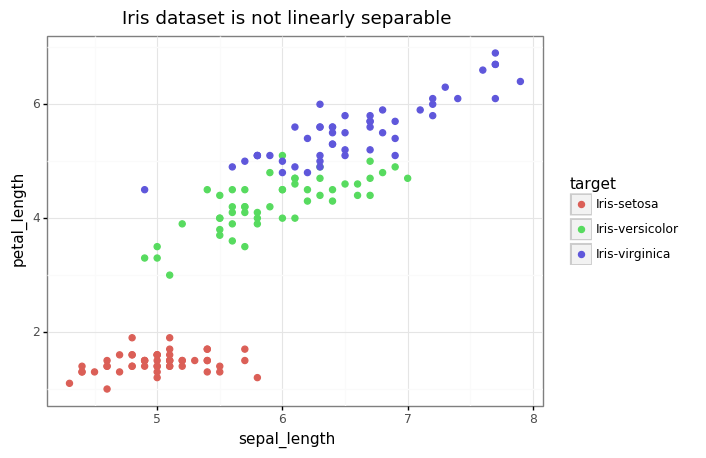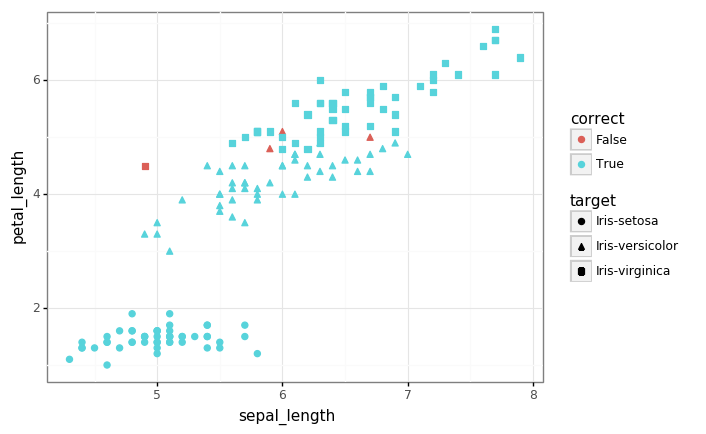In the spirit of our last post, let’s take things back to basics with a simple end-to-end data analysis example.

# Back to basics with pandas, plotnine, and sklearn

In this colab we do a quick review of the ‘data science basics’:

• Use pandas to store and manipulate the data.
• Use plotnine to generate plots/visualizations.
• Use scikit-learn to fit a simple logistic regression model.
# @title Imports

import numpy as np
import pandas as pd
import plotnine as gg
import requests
import sklearn


## Let there be data

Let’s fetch the famous Iris dataset from the UCI machine learning datasets repository.

# The URL for the raw data in text form.
data_url = 'https://archive.ics.uci.edu/ml/machine-learning-databases/iris/iris.data'

feature_columns = ['sepal_length', 'sepal_width', 'petal_length', 'petal_width']
target_columns = ['target']

# Fetch the raw data from the website.
response = requests.get(url=data_url)
rows = response.text.split('\n')
data = [r.split(',') for r in rows]

# Turn it into a dataframe and drop any null values.
df = pd.DataFrame(data, columns=feature_columns + target_columns)
df.dropna(inplace=True)

# Fix up the data types: features are numeric, targets are categorical.
df[feature_columns] = df[feature_columns].astype('float')
df[target_column] = df[target_column].astype('category')


## Take a quick look at the data

# Look at the raw data to check it looks sensible.

sepal_length sepal_width petal_length petal_width target
0 5.1 3.5 1.4 0.2 Iris-setosa
1 4.9 3.0 1.4 0.2 Iris-setosa
2 4.7 3.2 1.3 0.2 Iris-setosa
3 4.6 3.1 1.5 0.2 Iris-setosa
4 5.0 3.6 1.4 0.2 Iris-setosa
# Look at some summary statistics.
df.describe()

sepal_length sepal_width petal_length petal_width
count 150.000000 150.000000 150.000000 150.000000
mean 5.843333 3.054000 3.758667 1.198667
std 0.828066 0.433594 1.764420 0.763161
min 4.300000 2.000000 1.000000 0.100000
25% 5.100000 2.800000 1.600000 0.300000
50% 5.800000 3.000000 4.350000 1.300000
75% 6.400000 3.300000 5.100000 1.800000
max 7.900000 4.400000 6.900000 2.500000
# 6.1 KB ... now that's some #bigdata ;)
df.info()

<class 'pandas.core.frame.DataFrame'>
Int64Index: 150 entries, 0 to 149
Data columns (total 5 columns):
sepal_length    150 non-null float64
sepal_width     150 non-null float64
petal_length    150 non-null float64
petal_width     150 non-null float64
target          150 non-null category
dtypes: category(1), float64(4)
memory usage: 6.1 KB

# Let's take a visual look at the data now, projecting down to 2 dimensions.
gg.theme_set(gg.theme_bw())

p = (gg.ggplot(df)
+ gg.aes(x='sepal_length', y='petal_length', color='target')
+ gg.geom_point(size=2)
+ gg.ggtitle('Iris dataset is not linearly separable')
)
p## Fitting a simple linear model

Let’s get ‘old school’ and fit a simple logistic regression classifier using the raw features.

In the binary case, this is a linear probabilistic model with Bernoulli likelihood given by

$\mathcal{L}\left(\pmb{x}, y \lvert \pmb{x}\right) = f(\pmb{x})^y (1 - f(\pmb{x}))^{1-y},$

where $$f(\pmb{x}) = \sigma\left(\pmb{w}^T\pmb{x}\right)$$.

Assuming the dataset $$\mathcal{D}=\left\{\pmb{x}^{(i)}, y^{(i)}\right\}_{i=1}^{n}$$ is iid, the negative log-likelihood yields the familiar cross-entropy loss:

\begin{aligned} \log\mathcal{L}\left(\mathcal{D} \lvert \pmb{w}\right) &= \log\prod_{i=1}^{n} \sigma\left(\pmb{w}^T\pmb{x}^{(i)}\right)^{y^{(i)}} \left(1 - \sigma\left(\pmb{w}^T\pmb{x}^{(i)}\right)\right)^{1-y^{(i)}} \\ &= \sum_{i=1}^n y^{(i)}\log f\left(\pmb{x}^{(i)}\right) + (1-y^{(i)})\log\left(1 - f\left(\pmb{x}^{(i)}\right)\right) \end{aligned}

This is a convex and trivially differnetiable objective that we can optimize with gradient-based methods. We’ll defer this to scikit-learn (which also does some other tricks + regularization).

# Create a logistic regression model.
model = sklearn.linear_model.LogisticRegression(solver='lbfgs',
multi_class='multinomial')

# Extract the features and targets as numpy arrays.
X = df[feature_columns].values
y = df[target_column].cat.codes  # Note that we 'code' the categories as integers.

# Fit the model.
model.fit(X, y)

# Compute the model accuracy over the training set (we don't have a test set).
pred_df = df.copy()
pred_df['prediction'] = model.predict(X).astype('int8')
pred_df['correct'] = pred_df.prediction == pred_df.target.cat.codes
accuracy = pred_df.correct.sum() / len(pred_df)
print('Accuracy: {:.3f}'.format(accuracy))

Accuracy: 0.973

# Let's look again at the data, highlighting the examples which we mis-classified.
p = (gg.ggplot(pred_df)
+ gg.aes(x='sepal_length', y='petal_length', shape='target', color='correct')
+ gg.geom_point(size=2)
)
p